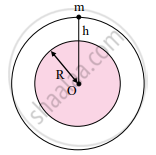# Answer the following question in detail. Show that acceleration due to gravity at height h above the Earth’s surface is gh=g(RR + h)2 and Discuss the variation of acceleration due to - Physics

Answer the following question in detail.

Show that acceleration due to gravity at height h above the Earth’s surface is "g"_"h" = "g"("R"/"R + h")^2

Answer the following question in detail.

Discuss the variation of acceleration due to gravity with altitude.

#### Solution

1. Let,
R = radius of the Earth,
M = mass of the Earth.
g = acceleration due to gravity at the surface of the Earth.
2. Consider a body of mass m on the surface of the Earth. The acceleration due to gravity on the Earth’s surface is given by,
"g" = "GM"/"R"^2     ....(1)3. The body is taken at height h above the surface of the Earth as shown in the figure. The acceleration due to gravity now changes to,
"g"_"h" = "GM"/("R + h")^2     .....(2)
4. Dividing equation (2) by equation (1), we get,
"g"_"h"/"g" = ("GM"/("R + h")^2)/("GM"/"R"^2)
therefore "g"_"h"/"g" = "R"^2/("R + h")^2
therefore "g"_"h" = "gR"^2/("R + h")^2
We can rewrite,
therefore "g"_"h" = "gR"^2/("R"^2(1 + "h"/"R")^2)
therefore "g"_"h" = "g"(1 + "h"/"R")^-2
5. For small altitude h, i.e., for "h"/"R" << 1, by neglecting higher power terms of "h"/"R", "g"_"h" = "g"(1 - "2h"/"R")

This expression can be used to calculate the value of g at height h above the surface of the Earth as long as h << R.

Concept: Variation in the Acceleration Due to Gravity with Altitude, Depth, Latitude and Shape
Is there an error in this question or solution?

#### APPEARS IN

Balbharati Physics 11th Standard Maharashtra State Board
Chapter 5 Gravitation
Exercises | Q 3. (iii) | Page 98
Balbharati Physics 11th Standard Maharashtra State Board
Chapter 5 Gravitation
Exercises | Q 3. (xvi) | Page 98